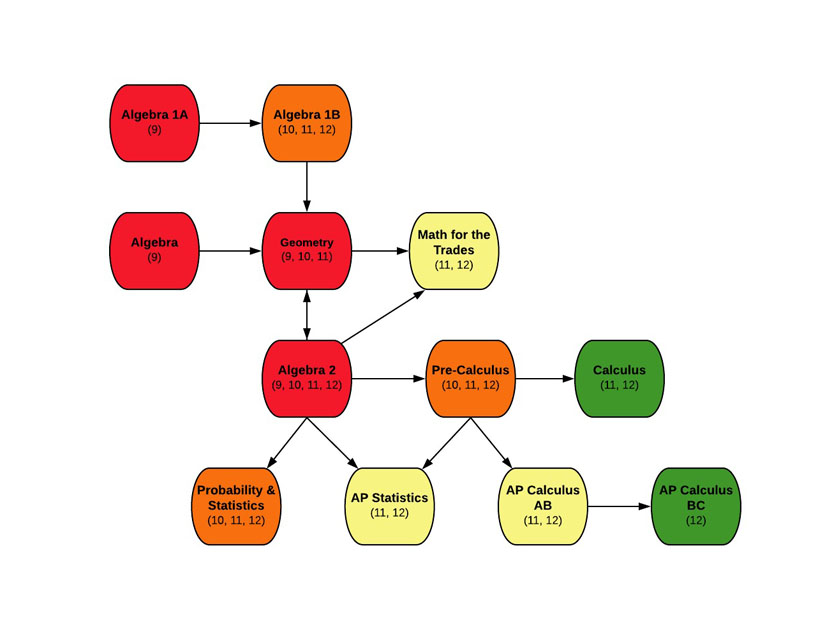EDIT MAIN11, 12

Mathematics, Transcripted Credit Courses

Accelerated, College Credit

1

This is a year long course that requires selection of both semesters: S1 and S2. Students will review and strengthen their basic computational skills with all types of numbers. Many areas of mathematics will be explored including Algebra, Geometry, Trigonometry and Statistics as they relate to the technical trades. Practical applications and real life applications of mathematics in the trade industries will be learned. Students will apply useful equations and formulas in real life problem solving situations.

Outcomes:

• Perform operations on whole numbers, fractions, decimals, and signed numbers
• Use proportions and percents to solve appropriate real-world problems
• Use English and Metric units of measure and tools such as rulers, micrometers, and calipers
• Perform unit conversions
• Solve one-step and multi-step equations and formulas
• Convert numbers from standard to scientific notation and back
• Calculate perimeter, circumference, and area of polygons and circles
• Calculate volume and surface area of 3-dimensional figures
• Use trigonometry and Pythagorean theorem to solve right triangles
• Solve systems of equations